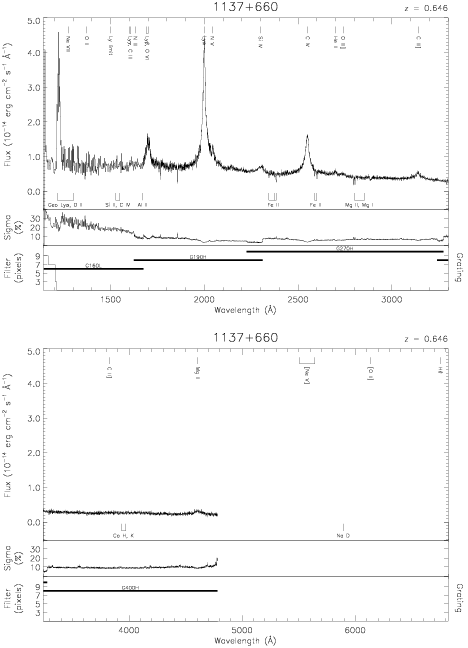Download dataset
TELESCOP= 'HST     '
INSTRUME= 'FOS     '
RADECSYS= 'FK5     '
EQUINOX =               2000.0
DATE-OBS= '1991-11-06T03:58:29'
RA_PNT  =       174.9878805668
DEC_PNT =        65.7970615722
PA_PNT  =       285.4909553576
OBJECT  = '1137+660'
ROOTNM01= 'Y0PE0902T'
GRNDMD01= 'SPECTROSCOPY'
DETECT01= 'AMBER   '
APERID01= 'A-1     '
FGWAID01= 'H19     '
ROOTNM02= 'Y0PE0903T'
GRNDMD02= 'SPECTROSCOPY'
DETECT02= 'AMBER   '
APERID02= 'A-1     '
FGWAID02= 'H27     '
ROOTNM03= 'Y0PE0904T'
GRNDMD03= 'SPECTROSCOPY'
DETECT03= 'AMBER   '
APERID03= 'A-1     '
FGWAID03= 'H40     '
ROOTNM04= 'Y0RV1H03T'
GRNDMD04= 'RAPID-READOUT'
DETECT04= 'AMBER   '
APERID04= 'C-2     '
FGWAID04= 'H19     '
ROOTNM05= 'Y0RV1H04T'
GRNDMD05= 'RAPID-READOUT'
DETECT05= 'AMBER   '
APERID05= 'C-2     '
FGWAID05= 'H27     '
ROOTNM06= 'Y0XX0602T'
GRNDMD06= 'RAPID-READOUT'
DETECT06= 'BLUE    '
APERID06= 'B-3     '
FGWAID06= 'L15     '